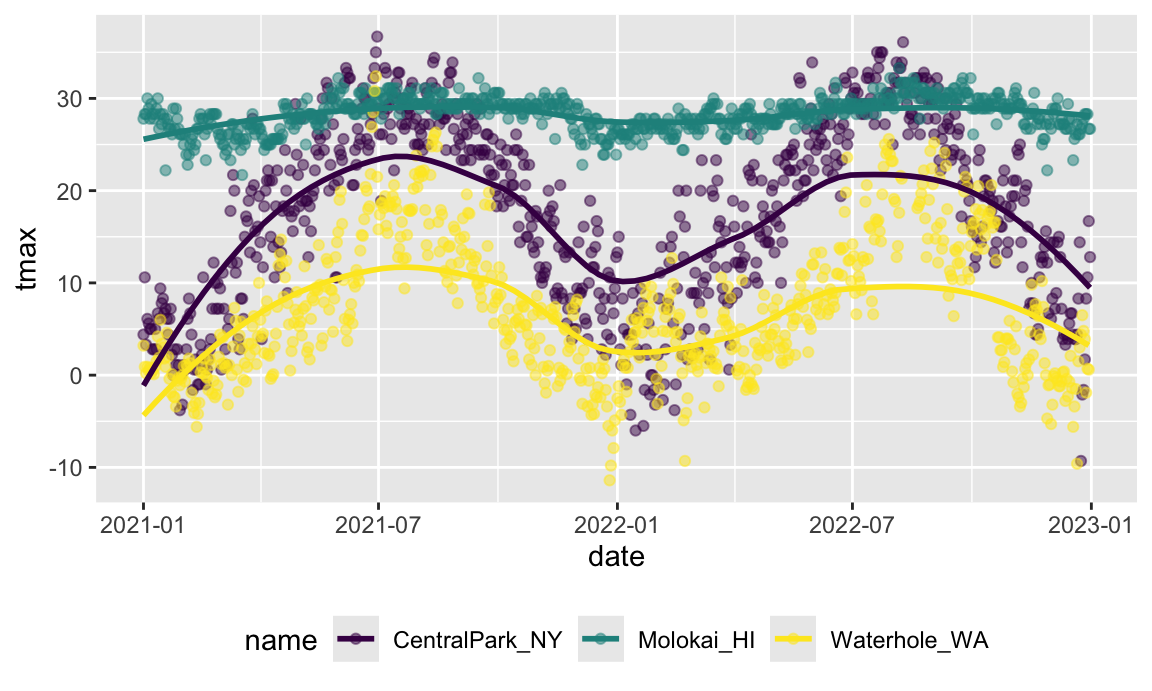In this, we’ll provide a basic definition of “data science” and discuss the connotation of the term in several contexts.

Overview

Learning Objectives

Define “data science” and understand its vital role in public health research.

Example

For the purpose of this class, we’ll use the following working definition of data science:

Data science is the use of data to formulate and rigorously answer questions in a process that emphasizes clarity, reproducibility, and collaboration, and that recognizes code as a primary means of communication.

In coming modules, we’ll learn about wrangling data, making visualizations, and conducting analyses. Throughout, we’ll focus on modern tools that facilitate best practices for working with data, including organization, reproducibility, and clear coding. Material will be presented in a way that combines didactic content with hands-on coding elements. Below are two examples we’ll return to later in the course.

Before introducing these, I’ll load the tidyverse.

library(tidyverse)
## ── Attaching packages ─────────────────────────────────────────────────────────────────────── tidyverse 1.3.0 ──
## ✓ ggplot2 3.3.0     ✓ purrr   0.3.4
## ✓ tibble  3.0.1     ✓ dplyr   1.0.2
## ✓ tidyr   1.0.2     ✓ stringr 1.4.0
## ✓ readr   1.3.1     ✓ forcats 0.5.0
## ── Conflicts ────────────────────────────────────────────────────────────────────────── tidyverse_conflicts() ──

The next chunk of code loads and tidies an example dataset, which includes daily record of several weather-related variables at each of three weather stations.

weather_df =
rnoaa::meteo_pull_monitors(
c("USW00094728", "USC00519397", "USS0023B17S"),
var = c("PRCP", "TMIN", "TMAX"),
date_min = "2017-01-01",
date_max = "2017-12-31") %>%
mutate(
name = recode(
id,
USW00094728 = "CentralPark_NY",
USC00519397 = "Waikiki_HA",
USS0023B17S = "Waterhole_WA"),
tmin = tmin / 10,
tmax = tmax / 10) %>%
select(name, id, everything())

As we’ll discuss, a major element of working with data is producing visualizations. The plot below shows the maximum temperature at each of the three stations, as well as smooth trends over time to illustrate seasonal effects. This is produced using ggplot, a package in the tidyverse that we’ll talk more about soon.

weather_df %>%
ggplot(aes(x = date, y = tmax, color = name)) +
geom_point(alpha = .5) +
geom_smooth(se = FALSE) +
theme(legend.position = "bottom")
## geom_smooth() using method = 'loess' and formula 'y ~ x'The next example uses data on Airbnb rentals in NYC, and is a bit more complex. The code below combines several steps to produce a map showing a sample of 5000 rentals in Brooklyn, Manhattan, and Queens; some important information (average rating, price, number of reviews) can be found by interacting with the map itself.

library(leaflet)
library(p8105.datasets)

data("nyc_airbnb")

nyc_airbnb =
nyc_airbnb %>%
mutate(stars = review_scores_location / 2) %>%
rename(boro = neighbourhood_group)

pal <- colorNumeric(
palette = "viridis",
domain = nyc_airbnb$stars) nyc_airbnb %>% filter(boro %in% c("Manhattan", "Brooklyn", "Queens")) %>% na.omit(stars) %>% sample_n(5000) %>% mutate( click_label = str_c("<b>$", price, "</b><br>", stars, " stars<br>", number_of_reviews, " reviews")) %>%
leaflet() %>%